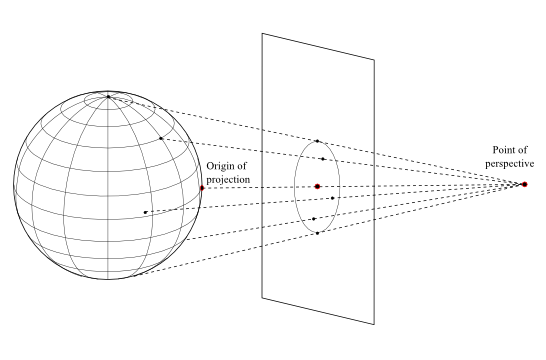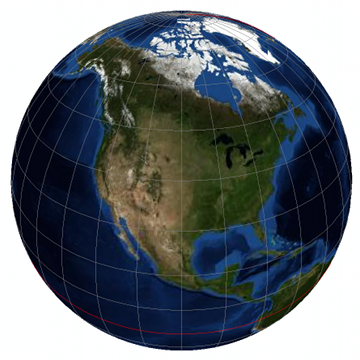2021.1.02

• All Implemented Interfaces:
ILcdGeneralPerspective, ILcdProjection, ILcdPropertyChangeSource, java.io.Serializable, java.lang.Cloneable

public class TLcdGeneralPerspective
extends ALcdGeneralPerspective
General perspective projection.

Introduction

The general perspective projection is an azimuthal projection using the origin of projection and the point of perspective. The origin height is taken into account. It is possible to tilt the projection plane.Properties

• The central meridian and a particular parallel (if shown) are projected as straight lines.
• Other meridians and parallels are usually arcs of circles or ellipses, but some may be parabolas or hyperbolas.
• Neither conformal (unless Stereographic), nor equal-area.
• If the point of perspective is above the center of the sphere or ellipsoid, les than one hemisphere may be shown (unless Orthographic).
• If the point of perspective is below the center of the globe, more than one hemisphere may be shown (cfr. Stereographic).
• There is considarable distortion near the projection limit.

ExampleSample code

ILcdProjection projection =
new TLcdGeneralPerspective(40.0, -100.0, 0.0, 2.0, 0.0, 0.0);

Implementation aspects

The equations of the general perspective projection are taken from [Snyder1987].

Note

The point of perspective has to be outside the earth ellipsoid. So, its value should be outside the closed interval [-1.0,1.0].

Serialized Form
• Constructor Detail

• TLcdGeneralPerspective

public TLcdGeneralPerspective()
Constructs a general perspective projection with the origin at point (0.0, 0.0, 0.0), the perspective distance at 2.0, the azimuth set to 0.0, and the tilt set to 0.0.
• TLcdGeneralPerspective

public TLcdGeneralPerspective(double aLongitude,
double aLatitude,
double aHeight,
double aPerspectiveDistance,
double aAzimuth,
double aTiltAngle)
Constructs a general perspective projection with the origin at a point (aLatitude, aLongitude, aHeight).
Parameters:
aLongitude - longitude of the origin of the projection, in decimal degrees.
aLatitude - latitude of the origin of the projection, in decimal degrees.
aHeight - height of the origin point above the ellipsoid.
aPerspectiveDistance - distance of the point of perspective from the center of the earth globe divided by the semi-major axis.
aAzimuth - azimuth east of north of the Y-axis of the Y_t axis of the tilted plane of projection
aTiltAngle - upward angle of tilt, or the angle between the Y_t axis and the tangent plane, expressed in degrees
• TLcdGeneralPerspective

public TLcdGeneralPerspective(ILcdPoint aOrigin,
double aPerspectiveDistance)
Constructs a general perspective projection with the origin at an ILcdPoint aOrigin and a point of perspective at a distance (divided by the semi-major axis) from the center of the earth. The properties azimuth and tiltAngle are set to zero.
Parameters:
aOrigin - point of origin (3D) of the projection.
aPerspectiveDistance - distance of the point of perspective from the center of the earth globe divided by the semi-major axis.
• TLcdGeneralPerspective

public TLcdGeneralPerspective(ILcdPoint aOrigin,
double aPerspectiveDistance,
double aAzimuth,
double aTiltAngle)
Constructs a general perspective projection with the origin at an ILcdPoint aOrigin and a point of perspective at a distance (divided by the semi-major axis) from the center of the earth.
Parameters:
aOrigin - point of origin (3D) of the projection.
aPerspectiveDistance - distance of the point of perspective from the center of the earth globe divided by the semi-major axis.
aAzimuth - azimuth east of north of the Y-axis of the Y_t axis of the tilted plane of projection
aTiltAngle - upward angle of tilt, or the angle between the Y_t axis and the tangent plane, expressed in degrees
• Method Detail

• calculateCachedValues

protected void calculateCachedValues()
Description copied from class: ALcdGeneralPerspective
Constants based on the longitude, latitude, height of the point of origin of projection and the distance of the point of perspective don't have to be recalculated every time a method is called. They only have to be calculated when the projection properties change. Within the methods the precalculated values then can be used in order to gain efficiency.
Overrides:
calculateCachedValues in class ALcdGeneralPerspective
• isAllInBounds

public boolean isAllInBounds()
Description copied from interface: ILcdProjection
If all geodetic coordinates (l ,j ) are projected (visible) by an ILcdProjection and all (x,y ) coordinates are bounded this is true. All ILcdProjection objects have bounded (x,y ) coordinates because the projection is cut off if necessary.
Returns:
true if all LonLatPoints are within the bounds of the projection, false otherwise.
• lonlatheight2worldOnSphereSFCT

public void lonlatheight2worldOnSphereSFCT(ILcdPoint aLLHP,
ILcd2DEditablePoint aWorldPoint)
throws TLcdOutOfBoundsException
Description copied from interface: ILcdProjection
Transforms an ILcd2DEditablePoint on a sphere into the cartesian coordinate system of the projection.
Parameters:
aLLHP - point to be projected.
aWorldPoint - projected point as side effect.
Throws:
TLcdOutOfBoundsException - If the ILcdPoint aLLHP is not within the valid area of the projection (visible area), then this exception is thrown.
• world2lonlatOnSphereSFCT

public void world2lonlatOnSphereSFCT(ILcdPoint aXYPoint,
ILcd2DEditablePoint aLLPSFCT)
throws TLcdOutOfBoundsException
Description copied from interface: ILcdProjection
Transforms an ILcdPoint from the cartesian coordinate system of the projection into an ILcd2DEditablePoint onto a sphere.
Parameters:
aXYPoint - projected point for which the corresponding geodetic point is sought.
aLLPSFCT - resulting geodetic coordinate as side effect.
Throws:
TLcdOutOfBoundsException - If the ILcdPoint aXYPoint is not within the valid area of the projection (visible area), then this exception is thrown.
• world2lonlatOnEllipsoidSFCT

public void world2lonlatOnEllipsoidSFCT(ILcdPoint aXYPoint,
ILcdEllipsoid aEllipsoid,
ILcd2DEditablePoint aLLPSFCT)
throws TLcdOutOfBoundsException
Transforms a world point to a LonLatHeightPoint. The height, which is taken into account, is supposed to be available in the input/output parameter aLLPSFCT.
Specified by:
world2lonlatOnEllipsoidSFCT in interface ILcdProjection
Overrides:
world2lonlatOnEllipsoidSFCT in class ALcdProjection
Parameters:
aXYPoint - projected point for which the corresponding geodetic point is sought.
aEllipsoid - ellipsoid on which the geodetic coordinate is defined.
aLLPSFCT - resulting geodetic coordinate as side effect.
Throws:
TLcdOutOfBoundsException - If the ILcdPoint aXYPoint is not within the valid area of the projection (visible area), then this exception is thrown.
• inLonLatBounds

public boolean inLonLatBounds(ILcdPoint aLLP)
Description copied from interface: ILcdProjection
Checks if an ILcdPoint is inside the valid area of geodetic coordinates of the projection. The valid area is determined by the LonLatPoints that are visible and for which the corresponding cartesian coordinate is within the boundaries.
Parameters:
aLLP - ILcdPoint to be checked upon.
Returns:
true if valid, false if invalid.
• boundaryLons

public double[][] boundaryLons(double lat)
Description copied from class: ALcdProjection
Default implementation. To be redefined for specific projections.
Specified by:
boundaryLons in interface ILcdProjection
Overrides:
boundaryLons in class ALcdProjection
Parameters:
lat - latitude in decimal degrees and -90.0 <= aLat<= 90.0.
Returns:
the array of pairs of longitudes and longitudes, where the closed interval longitudes -> longitudes is in visible area and -180.0 <= lon[i] <= 180.0.
• boundaryLats

public double[][] boundaryLats(double lon)
Description copied from class: ALcdProjection
Default implementation. To be redefined for specific projections.
Specified by:
boundaryLats in interface ILcdProjection
Overrides:
boundaryLats in class ALcdProjection
Parameters:
lon - longitude in decimal degrees -180.0 <= lon <= 180.0 .
Returns:
the array of pairs of latitudes lats and lats, where the closed interval lats -> lats is in visible area and -90.0 <= lats[i] <= 90.0 .
• world2DEditableBoundsOnSphereSFCT

ILcd2DEditableBounds aWorldBound)
The projection is bounded when the perspective distance is outside the closed interval [-1,1]. Currently a perspective distance within this closed interval [-1,1] is not supported.
Parameters:
aWorldBound - the bounds of the projection in world coordinates as side effect.
• inWorldBoundsOnSphere

public boolean inWorldBoundsOnSphere(ILcdPoint aXYPoint,
Description copied from interface: ILcdProjection
Checks if a ILcdPoint is inside the valid cartesian area of the projection. The valid cartesian area is determined naturally by the projection bounds or by an artificial introduced boundary limit because some cartesian points are at infinity. The shape of the valid cartesian area is in many cases rectangular or circular although it might be more complex.
Parameters:
aXYPoint - point to be checked upon.
Returns:
true if valid, false if invalid.
• toString

public java.lang.String toString()
Returns the String representation of the general perspective projection.
Specified by:
toString in interface ILcdProjection
Overrides:
toString in class java.lang.Object
Returns:
the String representation of the general perspective projection.